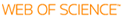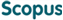### BROWSE

#### Related ResearcherLim, Sungbin
Research Interests

A SOBOLEV SPACE THEORY FOR STOCHASTIC PARTIAL DIFFERENTIAL EQUATIONS WITH TIME-FRACTIONAL DERIVATIVES

Cited 0 times inCited 0 times inTitle
A SOBOLEV SPACE THEORY FOR STOCHASTIC PARTIAL DIFFERENTIAL EQUATIONS WITH TIME-FRACTIONAL DERIVATIVES
Author
Issue Date
2019-07
Publisher
INST MATHEMATICAL STATISTICS
Citation
ANNALS OF PROBABILITY, v.47, no.4, pp.2087 - 2139
Abstract
In this article, we present an L-p-theory (p >= 2) for the semi-linear stochastic partial differential equations (SPDEs) of type partial derivative(alpha)(t)u = L(omega,t,x)u + f(u) + partial derivative(beta)(t) Sigma(infinity)(k=1)integral(t)(0)(Lambda(k)(omega,t,x)u + g(k)(u)) dw(t)(k), where alpha is an element of (0,2), beta < alpha + 1/2 and partial derivative(alpha)(t) and partial derivative(beta)(t) denote the Caputo derivatives of order alpha and beta, respectively. The processes omega(k)(t), k is an element of N={1,2, . . . }, are independent one-dimensional Wiener processes, L is either divergence or nondivergence-type second-order operator, and Lambda(k) are linear operators of order up to two. This class of SPDEs can be used to describe random effects on transport of particles in medium with thermal memory or particles subject to sticking and trapping. We prove uniqueness and existence results of strong solutions in appropriate Sobolev spaces, and obtain maximal L-p-regularity of the solutions. By converting SPDEs driven by d-dimensional space-time white noise into the equations of above type, we also obtain an L-p-theory for SPDEs driven by space-time white noise if the space dimension d < 4 - 2(2 beta -1)alpha(-1). In particular, if beta < 1/2 + alpha/4 then we can handle space-time white noise driven SPDEs with space dimension d = 1, 2, 3.
URI
https://scholarworks.unist.ac.kr/handle/201301/30829
URL
https://projecteuclid.org/euclid.aop/1562205704
DOI
10.1214/18-AOP1303
ISSN
0091-1798
Appears in Collections:
SME_Journal Papers
Files in This Item:
There are no files associated with this item.